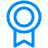Certificate

### Learn on Scaler Topics and get certified.# Fibonacci Series in Java

Learn about Fibonacci Series in Java using 4 methods

Updated - 13 Jun 20229 mins readPublished : 25 Aug 2021
Published : 25 Aug 2021Challenge Inside! : Find out where you stand! Try quiz, solve problems & win rewards!

## Overview

In this article, we will walk you through one of the most fundamental series in mathematics named fibonacci series. We will start with understanding the basic idea behind it and then we will learn the various types of implementation in Java for finding the nth fibonacci number.

## Scope

• In this article we will try to understand the fibonacci series.
• We will also try to understand how it can be implemented optimally in java.

## What is the Fibonacci Series?

It is a series of increasing numbers where the first two terms are 0 and 1 respectively. All other subsequent terms are obtained by adding the last two terms before them.

Mathematically,

Let f(n) returns us the nth fibonacci number

f(0)=0; f(1)=1;

f(n)=f(n-1)+f(n-2);

To calculate the Fibonacci number we have basic 2 approaches –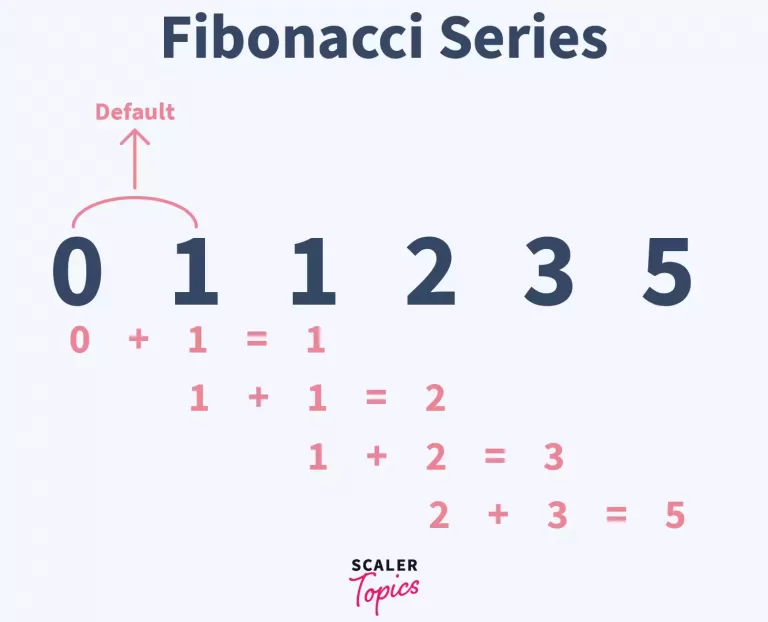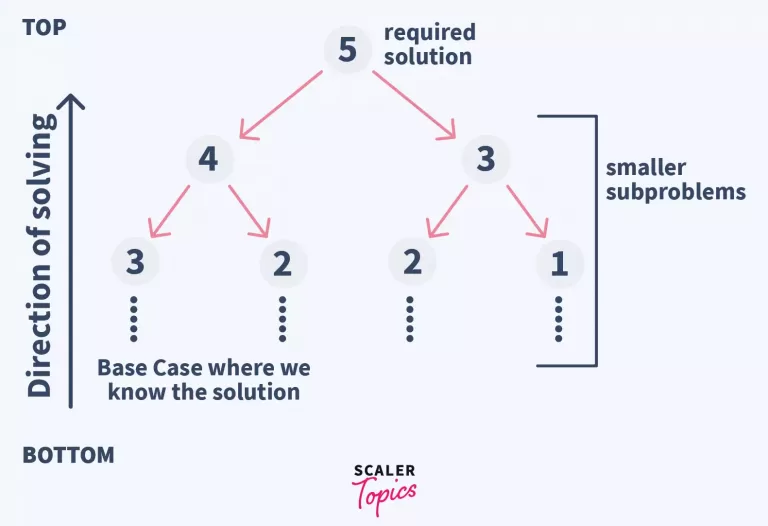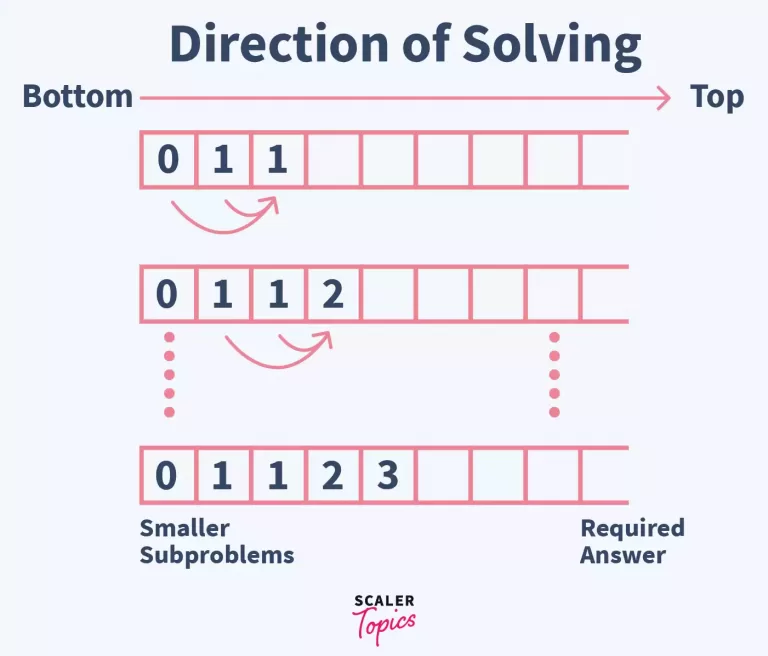• ### Top-Down Approach

Here we solve by building our solution for the top i.e., for the required index from smaller subproblems. We continue this process until we reach the point where we have some answer for our index.

From this point we start to build all the bigger answers until we find the answer for the required index. It is possible that we calculate one index multiple times.

• ### Bottom-Up Approach

Here we start from the smallest subproblems for which we have an answer. These subproblems help us find solutions to bigger subproblems. In this process all the possible cases from the smallest subproblem to the required problem are calculated.

## Program to Print Fibonacci Series in Java

### How do we approach it?

This method of problem-solving is called so because here we begin to find our solution by solving smaller subproblems first. These smaller subproblems later help us to find the bigger subproblems. We keep solving all the smaller subproblems until we are able to find our required solution.

Here our index keeps on increasing from smaller to bigger value.

Since we have to store all smaller answers before the required solution we create an array of sufficient size that can store all such values. Now we begin by storing the first 2 fibonacci numbers as 0 and 1 in this array.

values=0;values=1;

Now we start to find values for every remaining index. For this we start from the 3rd index. For all the indices we get their value by using the previous 2 values in the array as you can understand from the figure as well.

### Ex

```                            values=values+values;

values=values+values;
```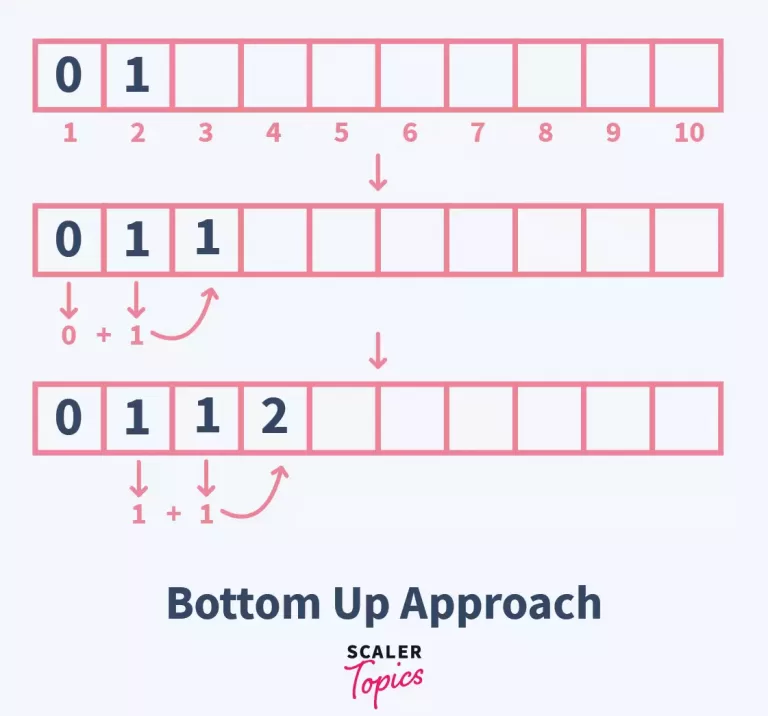### Program Algorithm Explanation

• Take input ‘n’ for determining the fibonacci number to be found

• Declare an array of size ‘n’ so that we can store all the values from first to nth fibonacci number

• Initialise the first 2 elements of the array with 0 and 1 respectively

• Run a loop from the 3rd element of the array

• For element at index ‘i’, we obtain their value by adding the most recent 2 values in the array using this statement

```                      values[i]=values[i-1]+values[i-2];
```
• Return the last element of the array i.e, the nth element

### Difficulties while programming this solution

• The array we created for storing the values of fibonacci numbers might not have sufficient memory to allocate the bigger fibonacci numbers. As you can see in the graph, the value of fibonacci numbers increases very quickly. This problem can be avoided if the ‘long long’ data type is used.
• The compiler might throw an error during runtime in declaring the array of size ‘n’ if ‘n’ is a very large number.

## Code for fibonacci numbers using For loop in Java

``````import java.util.*;
public class fibonacci{
public static void main(String args[]){
int n,i;
Scanner sc= new Scanner(System.in);
n=sc.nextInt();
sc.close();
int values[]=new int[n]; // space O(N) used
values=0;
values=1;
for(i=2;i<n;i++)values[i]=values[i-1]+values[i-2]; // single traversal O(N)
System.out.println("The nth fibonacci number is "+values[n-1]);
}
}
``````

## Code for Fibonacci Numbers Using While Loop in Java

``````import java.util.*;
public class fibonacci{
public static void main(String args[]){
int n,i;
Scanner sc= new Scanner(System.in);
n=sc.nextInt();
sc.close();
int values[]=new int[n]; // space O(N) used
values=0;
values=1;
i=2;
while(i<n) // single traversal O(N)
values[i]=values[i-1]+values[i-2];
i++;
System.out.println("The nth fibonacci number is "+values[n-1]);
}
}
``````

### Time Complexity : O(N) (Linear Time Complexity)

This is because for finding the nth fibonacci number we move through an array of size n( actually we are moving (n-2) indices in the array)

### Space Complexity: O(N) (Linear Space Complexity)

N -> index of nth fibonacci number

This is because for storing answers of all the smaller subproblems before the nth fibonacci number we need an array of size n.

### How do we approach it?

In this approach, we start solving from the required nth fibonacci number rather than solving all smaller subproblems. We try to solve only those subproblems that can give us the required solution. This method brings us from a bigger index to a smaller index.

For every fibonacci number, we look for 2 previous values in the series. If these values are not known to us we apply the same method for finding their solutions. Eventually, we will find our required index will keep on decreasing with time and we will reach the point where we already know the solution. Using this value we will keep on building all the values for indices that we came across before we hit the base case. At the end, we would have calculated our required answer.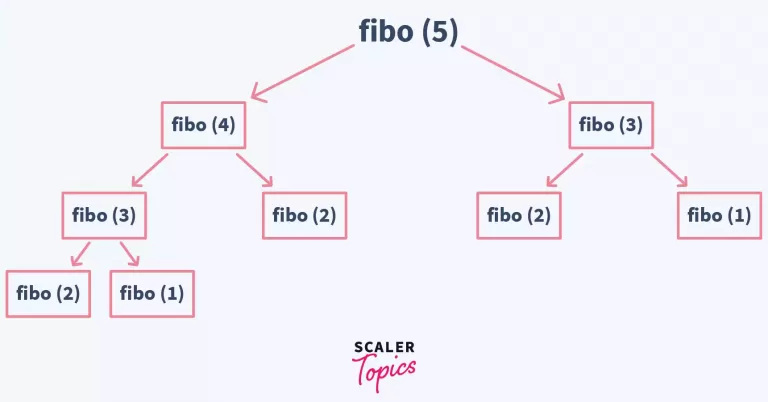## Code for fibonacci numbers using Recursion in Java

### Program Algorithm Explanation

• Here we use a custom user defined function fibo(used in the code) to find us the fibonacci number.
• In our main() we call our function fibo() for the nth fibonacci number.
• We expect this function to return us the nth fibonacci number.
• This function returns us 0 or 1 if we call it for 1st and 2nd fibonacci values.
• For all other values

Let fibo(x) returns xth fibonacci number

If x>2 then fibo(x)=fibo(x-1)+fibo(x-2)

• Here we move to the smaller parameters until we hit the base case and obtain some value.
• This program finds the value of many parameters more than once which increases our program’s runtime

### Difficulties while programming this solution

• This approach is although easy to understand but its time complexity is very poor. It can only be used if the required value’s index in series is small and ranges only up to 20.
• The functions in the program are stored on a function call stack whenever they are called. This call stack has a predefined limit for storing function calls. This stack throws an error whenever a lot of function calls exceeding its limit are pushed into it.

The above method is memory inefficient as it calculates already calculated values again. For this purpose it calls the same function multiple times to do the same thing and this throws an error for bigger values.

For reference while calculating 5th fibonacci value according to the above figure we are calculating 2nd fibonacci number 4 times.

``````import java.util.*;
public class fibonacci{
public static void main(String args[]){
int n;
Scanner sc= new Scanner(System.in);
n=sc.nextInt();
sc.close();
System.out.println(fibo(n));
}
public static int fibo(int n){
if(n==1)return 0;
else if(n==2)return 1;
else{
return fibo(n-1)+fibo(n-2);
}
}
}
``````

### Time Complexity : O(2^N) (Exponential Time Complexity)

N -> index of nth fibonacci number

Since every value is made up of previous 2 values we need to find 2 values for finding any fibonacci number. This gives rise to 2 calls everytime in our recursion tree. The tree can have at most n levels. This gives us at most 2^n nodes in the tree

### Space Complexity: O(2^N) (Exponential Space Complexity)

N -> index of nth fibonacci number

All the function calls are pushed in the function call stack. At any point of time at max 2^n function calls are there in call stack therefore space complexity will be O(2^n)

## Code for Fibonacci Numbers using Recursion with Memoization in Java

Memoization refers to storing all the solutions to smaller subproblems while solving a problem. This saves us time as we can then easily retrieve the stored value and we don’t need to calculate them again.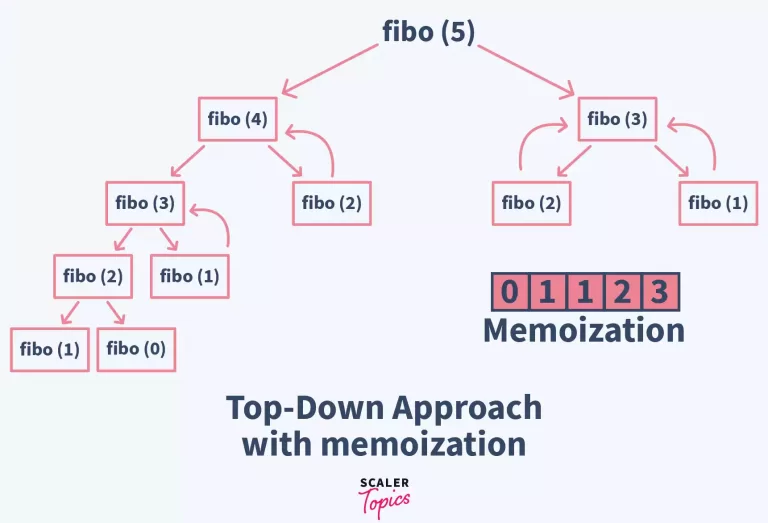### Program Algorithm Explanation

• Here we use a user defined function fibo(used in the code) to find us the fibonacci number.
• Here we declare a global array with a large size to accommodate fibonacci numbers once they are calculated.
• In our main() we call our function fibo() for the nth fibonacci number.
• We expect this function to return us the nth fibonacci number.
• This function returns us 0 or 1 if we call it for 1st and 2nd fibonacci values.
• For all other values

Let fibo(x) returns xth fibonacci number

If x>2 then fibo(x)=fibo(x-1)+fibo(x-2)

Till here it is similar to our program which implemented only recursion.

• Now for every value computed we store it in our global array values[].
• In values[] ith fibonacci number is stored at (i-1)th index.
• This helps us to reduce the complexity of our code to approximately O(N).

Since we already stored our pre-calculated values in the values[] array we use them to avoid the same function calls which have already been called. By this it overcomes one of the challenges of recursion without memorization.

``````import java.util.*;
public class fibonacci{
public static int fibo(int n){
if(n==1)return values;
if(n==2)return values;
else{
values[n-1]=fibo(n-1)+fibo(n-2);
return values[n-1];
}
}
public static void main(String args[]){
int n;
Scanner sc= new Scanner(System.in);
n=sc.nextInt();
sc.close();
values=0;
values=1;
System.out.println(fibo(n));
}
static int values[]=new int;
}
``````

### Time Complexity : O(N) (Linear Time Complexity

N -> index of nth fibonacci number

In this method at each ith level of the recursion tree 2^i nodes must have been added for computation just like normal recursion. But since we store every pre-computed value(memoization), we only solve for new indices.

### Space Complexity: O(N) (Linear Space Complexity

N -> index of nth fibonacci number

Since only n indices are being computed only their function calls are entered in stack memory.

## Conclusion

Hence we studied the fibonacci series and its implementation in java using 4 ways –

• Bottom-Up Approach
• Using For Loop
• Using While Loop
• Top-Down Approach
• Using Recursion with memoization
• Using Recursion without memoization

After studying all the methods to find the fibonacci numbers we can come to a conclusion that using recursion with memoization is the best method for solving here. It provides us with the best possible time complexity and space complexity.

### Things to remember while solving for fibonacci numbers –

• Ensure your data type is sufficiently big enough to accommodate your fibonacci values
• While using the top down approach in recursion ensure that you use memoization by storing every value calculated at runtime
Challenge Time!Time to test your skills and win rewards! Note: Rewards will be credited after the next product update.
Free Courses by top Scaler instructors